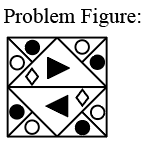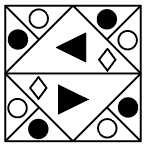Question 4

# Which of the option figures is the exact mirror image of the given problem figure when the mirror is held to the right side of the problem figure?Solution

Given imageFrom the given image mirror concept held to the right side of the problem figuretherefore( B)Ans